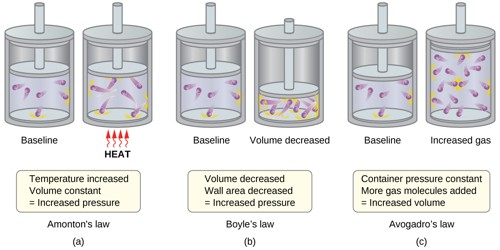# Fundamental Postulates of Gas Molecules

Fundamental Postulates of Gas Molecules

Heat is generated due to the motion of gas molecules. It is the kinetic theory of gases. From the kinetic theory of gases the nature of gas and the relation between generated heat can be found. In order to establish kinetic theory of gases some preconditions are called fundamental postulates. These fundamental postulates of gas molecules are mentioned below:

1. Each gas is compared of innumerable tiny particles of equal masses. These are called molecules. Molecules follow Newton’s laws of motion.
2. Molecules of any gas are identical. But molecules of different gases are different. For example— all molecules of hydrogen gas are alike, all molecules of oxygen gas are alike. But molecules of hydrogen gas and Molecules of oxygen gas are not alike.
3. Molecules of gases are considered as ideal elastic spheres.
4. Volume of the molecules is negligible compared to the distance between molecules.
5. Total volume of the molecules is exceedingly small compared to the volume of the container.
6. There does not exist any attractive or repulsive force between molecules or the molecules do not assert force on the walls of the container. That means, energy of the molecules is kinetic energy.
7. Molecules are always in motion; their velocities may be extended from zero to infinity.
8. Molecules are in a state of continuous random motion in all directions and are colliding with each other and also with the walls of the container. Pressure is generated due to the collision of the molecules with the walks.
9. Velocity of molecules increases with the increase of temperature.
10. Between two collisions molecules travel in straight line. Distance between two collisions is called mean free path.
11. Time taken for one collision is exceedingly small compared to the time taken to travel the mean free path, hence collisions are considered as instantaneous.
12. Gas molecules are able to move freely all over the volume of the container.
13. Even though there are continuous collisions between molecules, but number of molecules per unit volume remains constant. From this it can be concluded that the atomic density of ideal gas always remains constant. Here it is to be mentioned that a gas which obeys all the fundamental postulates of gas is called an ideal gas.
14. All the collisions are instantaneous.
15. Absolute temperature of the gas is proportional to the total kinetic energy of the molecules.